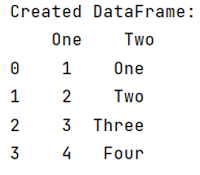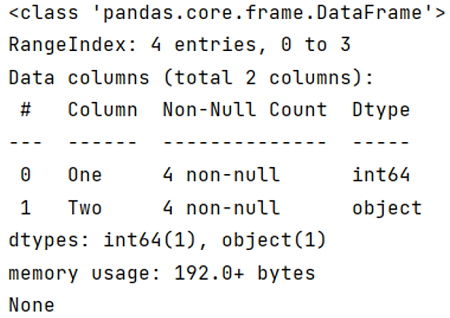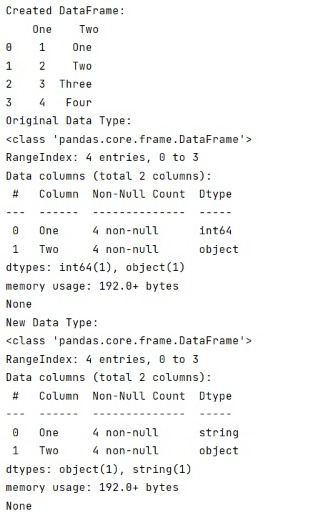# How to convert column value to string in pandas DataFrame?

Given a DataFrame, we need to convert a column value which is not of string type into string data type. By Pranit Sharma Last updated : September 20, 2023

A string is a group of characters. A string can contains any type of character including numerical characters, alphabetical characters, special characters, etc.

## Problem statement

Given a DataFrame, we need to convert a column value which is not of string type into string data type.

## Converting column value to string in pandas DataFrame

To check the data type of each column in a DataFrame, we use pandas.DataFrame.info() method which tells us about every piece of information of the DataFrame, and to convert column value to string, we use pandas.DataFrame.astype() with the specified column by passing the data type inside the function.

Syntax of pandas.DataFrame.info() Method:

```DataFrame.info(
verbose=None,
buf=None,
max_cols=None,
memory_usage=None,
show_counts=None,
null_counts=None
)
```
Note

To work with pandas, we need to import pandas package first, below is the syntax:

```import pandas as pd
```

## Create a Pandas DataFrame

```# Importing Pandas package
import pandas as pd

# Create a dictionary
d = {
'One':[1,2,3,4],
'Two':['One','Two','Three','Four']
}

# Create DataFrame
df = pd.DataFrame(d)

# Display DataFrame
print("Created DataFrame:\n",df)
```

The output of the above program is:## Check and print the data type of Pandas DataFrame

Now let's understand how pandas.DataFrame.info() works?

```# Display df.info
print(df.info())
```

The output of the above program is:## Convert column value to string in pandas DataFrame

We can observe that the values of column 'One' is an int, we need to convert this data type into string or object.

For this purpose we will use pandas.DataFrame.astype() and pass the data type inside the function.

Let us understand with the help of an example,

```# Importing Pandas package
import pandas as pd

# Create a dictionary
d = {
'One':[1,2,3,4],
'Two':['One','Two','Three','Four']
}

# Create DataFrame
df = pd.DataFrame(d)

# Display DataFrame
print("Created DataFrame:\n",df)

# Display df.info
print("Original Data Type:\n",df.info())

# Converting column One values into string type
df['One'] = df['One'].astype('string')

# Display df.info
print("New Data Type:\n",df.info())
```

The output of the above program is: